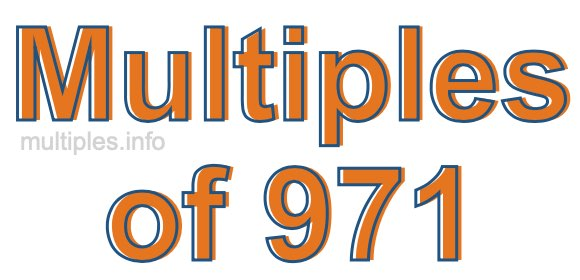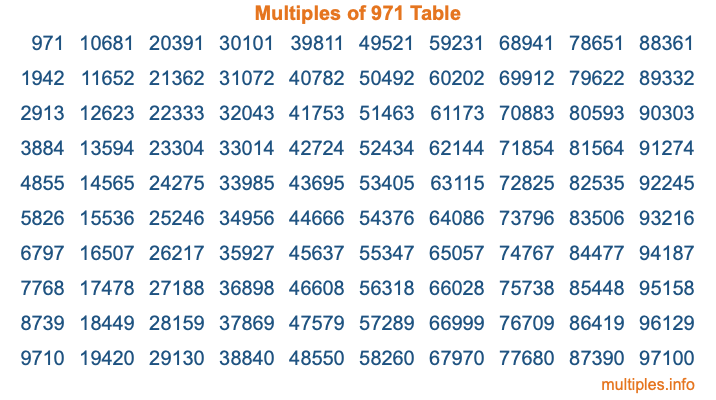Multiples of 971Welcome to the Multiples of 971 page. Here we will first teach you everything you will ever need to know about the multiples of 971, and then give you a study guide summary of everything we taught you to make sure you remember it all. Use this page to look up facts and learn information about the multiples of 971. This page will make you a multiples of nine hundred seventy-one expert!

Definition of Multiples of 971
Multiples of 971 are all the numbers that when divided by 971 equal an integer. Each of the multiples of 971 are called a multiple. A multiple of 971 is created by multiplying 971 by an integer.

Therefore, to create a list of multiples of 971, you start with 1 multiplied by 971, then 2 multiplied by 971, then 3 multiplied by 971, and so on for as long as you want. Thus, the list of the first five multiples of 971 is 971, 1942, 2913, 3884, and 4855. To see a larger list of multiples of 971, see the printable image of Multiples of 971 further down on this page. We also have a category where you can choose any nth multiple of 971.

Multiples of 971 Checker
The Multiples of 971 Checker below checks to see if any number of your choice is a multiple of 971. In other words, it checks to see if there is any number (integer) that when multiplied by 971 will equal your number. To do that, we divide your number by 971. If the the quotient is an integer, then your number is a multiple of 971.

Is  a multiple of 971?

Least Common Multiple of 971 and ...
A Least Common Multiple (LCM) is the lowest multiple that two or more numbers have in common. This is also called the smallest common multiple or lowest common multiple and is useful to know when you are adding our subtracting fractions. Enter one or more numbers below (971 is already entered) to find the LCM.

Check out our LCM Calculator if you need more details about the Least Common Multiple or if you need the LCM for different numbers for adding and subtraction fractions.

nth Multiple of 971
As we stated above, 971 is the first multiple of 971, 1942 is the second multiple of 971, 2913 is the third multiple of 971, and so on. Enter a number below to find the nth multiple of 971.

th multiple of 971

Multiples of 971 vs Factors of 971
971 is a multiple of 971 and a factor of 971, but that is where the similarities end. All postive multiples of 971 are 971 or greater than 971. All positive factors of 971 are 971 or less than 971.

Below is the beginning list of multiples of 971 and the factors of 971 so you can compare:

Multiples of 971: 971, 1942, 2913, 3884, 4855, etc.

Factors of 971: 1, 971

As you can see, the multiples of 971 are all the numbers that you can divide by 971 to get a whole number. The factors of 971, on the other hand, are all the whole numbers that you can multiply by another whole number to get 971.

It's also interesting to note that if a number (x) is a factor of 971, then 971 will also be a multiple of that number (x).

Multiples of 971 vs Divisors of 971
The divisors of 971 are all the integers that 971 can be divided by evenly. Below is a list of the divisors of 971.

Divisors of 971: 1, 971

The interesting thing to note here is that if you take any multiple of 971 and divide it by a divisor of 971, you will see that the quotient is an integer.

Multiples of 971 Table
Below is an image of the first 100 multiples of 971 in a table. The table is in chronological order, column by column. The first column has the first ten multiples of 971, the second column has the next ten multiples of 971, and so on.The Multiples of 971 Table is also referred to as the 971 Times Table or Times Table of 971. You are welcome to print out our table for your studies.

Negative Multiples of 971
Although not often discussed or needed in math, it is worth mentioning that you can make a list of negative multiples of 971 by multiplying 971 by -1, then by -2, then by -3, and so on, to get the following list of negative multiples of 971:

-971, -1942, -2913, -3884, -4855, etc.

Multiples of 971 Summary
Below is a summary of important Multiples of 971 facts that we have discussed on this page. To retain the knowledge on this page, we recommend that you read through the summary and explain to yourself or a study partner why they hold true.

There are an infinite number of multiples of 971.

A multiple of 971 divided by 971 will equal a whole number.

971 divided by a factor of 971 equals a divisor of 971.

The nth multiple of 971 is n times 971.

The largest factor of 971 is equal to the first positive multiple of 971.

971 is a multiple of every factor of 971.

971 is a multiple of 971.

A multiple of 971 divided by a divisor of 971 equals an integer.

971 divided by a divisor of 971 equals a factor of 971.

Any integer times 971 will equal a multiple of 971.

Multiples of a Number
Here you can get the multiples of another number, all with the same attention to detail as we did for multiples of 971 on this page.

Multiples of
Multiples of 972
Did you find our page about multiples of nine hundred seventy-one educational? Do you want more knowledge? Check out the multiples of the next number on our list!